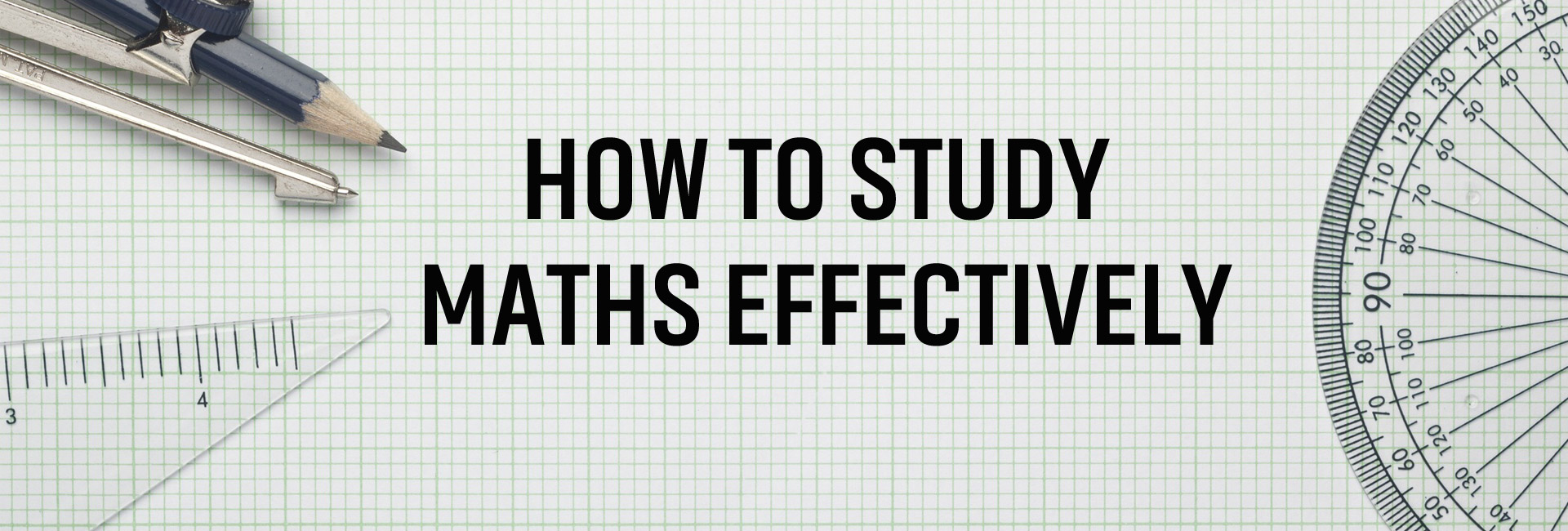Share

# How To Study Maths Effectively

All the subjects are important for the student, whether it is English, Science, Social Science, Hindi or even art. But Mathematics is considered as one of the vital subjects for students. This is a subject that students cannot avoid. Most students love to study maths, but some students hate this subject. The students who don’t like to study mathematics face a lot of stress at the time of homework, finding assignments, and especially on exams days. Mathematics is much different than the other subjects but only for those who don’t know the best way to study math.

## Here Are Some Ways to Study Maths Effectively.

### 1. Maths Need Practice

Mathematics is not a subject of only reading and listening. So when it comes to math, do more and more practice because when you practice or solve the problems of mathematics, it becomes easy to understand. The more you practice or solve the math problems, the better you can grab and understand it. Mathematics is all about formulas, identities, and calculations. So it is important to solve each problem of mathematics in numerous ways before your exam. To score good marks in mathematics, you need to solve a lot of mathematical problems because mathematics needs more and more practice.

When you are solving the mathematics problems and made any kind of mistakes, then you should review those mistakes and understand that where you are wrong. If you consider your mistake and understand that how to solve them, it will help you in the future to avoid the same kind of errors. This is the best way to become stronger in mathematics. So review your all the mistakes of mathematics that you made, solve at least two-three times to avoid the mistakes.

### 3. Remember The Key Concepts

Always remember the key concepts of mathematics instead of memorizing the whole process. While you are solving mathematics, at that time only focus on the key concepts. Understanding the logic and process used in the problems because it will help you in exams and the future. Mathematics is considered the sequential subject, so it is essential to have a dense understanding of the key concepts. As we know that mathematics is difficult to solve, so try to understand it’s all the formulas, methods, and identities.

### 4. Try to Understand Your Doubts

Many times students can get stuck when they are trying to solve the mathematics problems, and because of this, they are not able to move on the next step. Some students skip that question and continue the other one, but it’s not the right way to solve mathematics problems. Avoid skipping the mathematics problems, try to solve and understand the questions. Mathematics always needs patience and time to solve the problems. If you are not able to solve any complex question, then ask your teacher for help, they will definitely help you clear your doubts. You can also study with your friends to solve the mathematics problems and consult with them to solve complex problems.

### 5. Avoid Distraction

While studying mathematics, avoid all the distraction that becomes barriers to your study. Mathematics is a subject that needs full attentiveness than any other subject. This subject needs the study area, which is free of distraction. When you are studying the complex topics of mathematics like trigonometry, algebra, geometry, then make sure that no one can disturb you. While you are studying mathematics, you can also be listening to music to create a relaxing environment because background music can stimulate an environment, and you can concentrate more on your studies.

### 6. Make Notes

To study mathematics more effectively, make sure that you take all the notes during your lecture. Note down all things in detail so that you can understand all the points. When your teacher teaches you in class, you should understand every method and formula. The notes that you take in class can help you to do homework, and you can also recall the things that your teacher taught you in class. If you take the detailed notes and listen to the lecture carefully, then it will help you to solve any problem quickly.